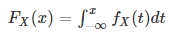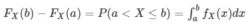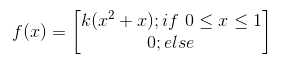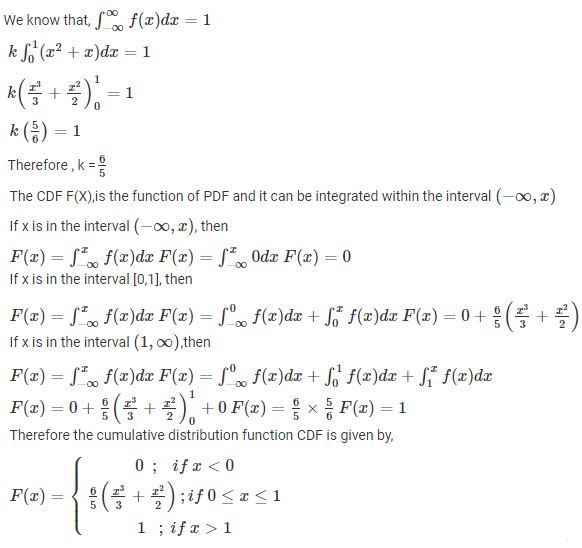# Cumulative Distribution Function

In Probability and Statistics, the Cumulative Distribution Function (CDF) of a real-valued random variable, say “X”, which is evaluated at x, is the probability that X takes a value less than or equal to the x. A random variable is a variable that defines the possible outcome values of an unexpected phenomenon. It is defined for both discrete and random variables. It is also used to specify the distribution of the multivariate random variables. If the random variable is above a particular level, it is known as tail distribution or the Complementary Cumulative Distribution Function (CCDF). In this article, you will understand what cumulative distribution function is, its properties, formulas, applications and examples.

## What is a Cumulative Distribution Function?

The Cumulative Distribution Function (CDF), of a real-valued random variable X, evaluated at x, is the probability function that X will take a value less than or equal to x. It is used to describe the probability distribution of random variables in a table. And with the help of these data, we can easily create a CDF plot in an excel sheet.

In other words, CDF finds the cumulative probability for the given value. To determine the probability of a random variable, it is used and also to compare the probability between values under certain conditions. For discrete distribution functions, CDF gives the probability values till what we specify and for continuous distribution functions, it gives the area under the probability density function up to the given value specified.

## Cumulative Distribution Function Formula

The CDF defined for a discrete random variable and is given as

Fx(x) = P(X ≤ x)

Where X is the probability that takes a value less than or equal to x and that lies in the semi-closed interval (a,b], where a < b.

Therefore the probability within the interval is written as

P(a < X ≤ b) = Fx(b) – Fx(a)

The CDF defined for a continuous random variable is given as;Here, X is expressed in terms of integration of its probability density function fx.

In case, if the distribution of the random variable X has the discrete component at value b,

P(X = b) = Fx(b) – limx→b- Fx(x)

## Cumulative Distribution Function Properties

The cumulative distribution function Fx(x) of a random variable has the following important properties:

• Every CDF Fx is non decreasing and right continuous

limx→-∞Fx(x) = 0 and limx→+∞Fx(x) = 1

• For all real numbers a and b with continuous random variable X, then the function fx is equal to the derivative of Fx, such that• If X is a completely discrete random variable, then it takes the values x1, x2, x3,…  with probability pi = p(xi), and the CDF of X will be discontinuous at the points xi:

FX(x) = P(X ≤ x) =

$$\begin{array}{l}\sum_{x_i\le x}P(X = x_i)=\sum_{x_i\le x}p(x_i)\end{array}$$

This function is defined for all real values, sometimes it is defined implicitly rather than defining it explicitly. The CDF is an integral concept of PDF ( Probability Distribution Function )

Consider a simple example for CDF which is given by rolling a fair six-sided die, where X is the random variable

We know that the probability of getting an outcome by rolling a six-sided die is given as:

Probability of getting 1 = P(X≤ 1 ) = 1 / 6

Probability of getting 2 = P(X≤ 2 ) = 2 / 6

Probability of getting 3 = P(X≤ 3 ) = 3 / 6

Probability of getting 4 = P(X≤ 4 ) = 4 / 6

Probability of getting 5 = P(X≤ 5 ) = 5 / 6

Probability of getting 6 = P(X≤ 6 ) = 6 / 6 = 1

From this, it is noted that the probability value always lies between 0 and 1 and it is non-decreasing and right continuous in nature.

### Cumulative Frequency Distribution

The set of data represented in a tabular or graphical form, showing the frequency of observations occurring in a given interval, is the frequency distribution. In the case of cumulative frequency, the number of observations that occur beyond any specific observation is calculated. To learn in detail, visit the article for cumulative frequency distribution and understand the concept thoroughly with the help of examples.

## Cumulative Distribution Function Applications

The most important application of cumulative distribution function is used in statistical analysis. In statistical analysis, the concept of CDF is used in two ways.

• Finding the frequency of occurrence of values for the given phenomena using cumulative frequency analysis.
• To derive some simple statistics properties, by using an empirical distribution function, that uses a formal direct estimate of CDFs.

### Cumulative Distribution Function Example

Question:

The random variable with PDF is given by:Find the cumulative distribution function(CDF)

Solution: The random variable with Probability Distribution Function is given to us. Let is find the CDF now;For more information about mathematics articles, solved problems and video tutorials register with BYJU’S – The Learning App.

## Frequently Asked Questions – FAQs

Q1

### What is meant by the cumulative distribution function?

The cumulative distribution function (CDF) of random variable X is defined as FX(x) = P(X ≤ x), for all x ∈ R. Note that the subscript X indicates that this is the CDF of the random variable X. Also, note that the CDF is defined for all x ∈ R.
Q2

### What does a CDF do?

The cumulative distribution function (CDF) calculates the cumulative probability for a given x-value. Use the CDF to determine the likelihood that a random observation taken from the population will be less than or equal to a particular value.
Q3

### What are PDF and CDF?

For a continuous function, the probability density function (PDF) is the probability that the variate has the value x. Since for continuous distributions, the probability at a single point is zero. Generally, this can be expressed in terms of integration between two points.
The Cumulative Distribution Function (CDF) of a real-valued random variable X, evaluated at x, is the probability function that X will take a value less than or equal to x. It is used to describe the probability distribution of random variables in a table. And with the help of these data, we can easily create a CDF plot in an excel sheet.
Q4

### What is the range of values of the cumulative distribution function?

The cumulative distribution function, FX(t) ranges from 0 to 1.
Q5

### Can cumulative distribution function greater than 1?

Only the integral of the density (i.e., the cumulative [probability] distribution function, C[P]DF) must be 1. We know that the cumulative distribution function, FX(t) ranges from 0 to 1.
Q6

### Is CDF always increasing?

A CDF can be either increasing or strictly increasing. A CDF will not be strictly increasing whenever there is a discontinuity in the set of values the variable can take on: for example, a discrete distribution.